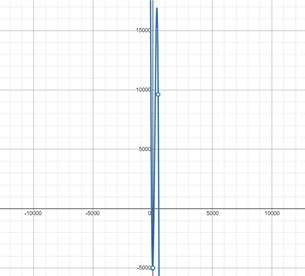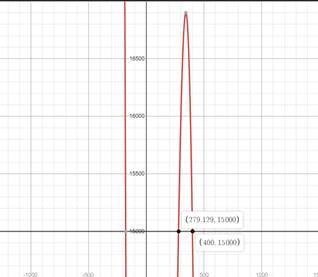# Graph the given equation in given interval.### Precalculus: Mathematics for Calcu...

6th Edition
Stewart + 5 others
Publisher: Cengage Learning
ISBN: 9780840068071### Precalculus: Mathematics for Calcu...

6th Edition
Stewart + 5 others
Publisher: Cengage Learning
ISBN: 9780840068071

#### Solutions

Chapter 1.9, Problem 72E

a.

To determine

## Graph the given equation in given interval.

Expert Solution### Explanation of Solution

Given information:

y=10x+0.5x20.001x350000x450

Making Graph of y=10x+0.5x20.001x35000b.

To determine

### Number of cooktops to be produced to begin generating profit

Expert Solution

So At least 100 cooktops should be generated to produce profit.

### Explanation of Solution

Given information:

y=10x+0.5x20.001x350000x450

Formula used:

The point where the graph intersect the x-axis is required solution.

The graph intersect the x-axis in interval of 0x450 at (100,0). So At least 100 cooktops should be generated to produce profit.

c.

To determine

Expert Solution

x(279.12,400)

### Explanation of Solution

Given information:

y=10x+0.5x20.001x350000x450

Formula used:

Plotting of Graph

As per the given condition, below is the required inequality:

150010x+0.5x20.001x35000

Making graph of y=10x+0.5x20.001x35000 and y=1500 in one viewing rectangle.The region where y=10x+0.5x20.001x35000 is above y=1500 is required solution.

x(279.12,400)

### Have a homework question?

Subscribe to bartleby learn! Ask subject matter experts 30 homework questions each month. Plus, you’ll have access to millions of step-by-step textbook answers!